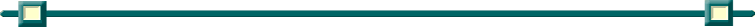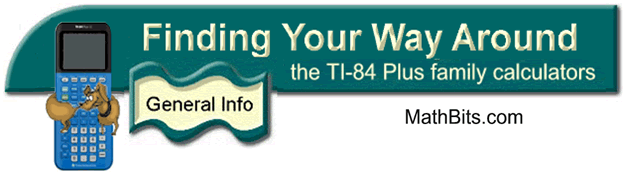Mode Key
 Hit the MODE key:
 Classic Mode: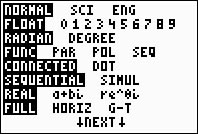MathPrint mode adds a second page: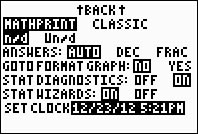CE model offers more options: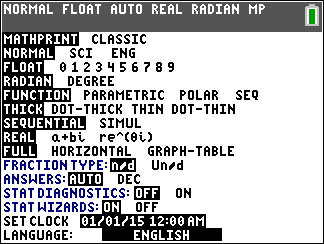The shaded choices are set by default (meaning they are turned on unless you change them). Notice that the MathPrint mode (if available) is turned on by default. You can change back to Classic mode, but you will need to move to the statement CLASSIC, and press ENTER to highlight (engage) that choice.

Available Options:

 Notation modes only affect the way answers are displayed on the Home screen. Numeric values can be displayed with up to 10 digits and a two-digit exponent.NORMAL notation displays digits to the left and right of the decimal point, as entered. It is the usual way we write numbers. In NORMAL mode, if the number is beyond 10 digits, or the absolute value is less than 0.001, the calculator will express the answer in scientific notation.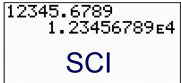SCI (scientific) notation displays one digit to the left of the decimal point, with the remaining significant digits to the right of the decimal with the appropriate power of 10 displayed to the right of E.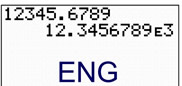ENG (engineering) notation displays the value with one, two, or three digits to the left of the decimal point, with the power of 10 exponent expressed in multiples of three.

 FLOAT (means floating decimal point) displays up to 10 digits plus the sign and the decimal point.
 0 1 2 3 4 5 6 7 8 9 (fixed decimal point) specifies the number of digits (from 0 to 9) to display to the right of the decimal point. Move the cursor to the desired number of decimal points and hit ENTER.

 FUNC (function):   plots functions with Y being a function of X. PAR (parametric):   plots relations with X and Y being functions of T. POL (polar):   plots functions with r being a function of theta. SEQ (sequence):   plots sequences
 CONNECTED: draws a line connecting each point calculated of the function. DOT: plots only the calculated points of the funciton.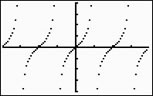SEQUENTIAL:   this choice evaluates and plots ONE function completely before the next function is evaluated and plotted.
SIMUL (simultaneous):   this choice evaluates and plots ALL functions for a single value of X and then evaluates and plots them ALL for the next value of X.

REAL:   complex numbers are not displayed unless entered as input.
a + bi:   (rectangular complex mode) complex numbers are displayed in a + bi form.
re^Øi:   (polar complex mode) complex numbers are displayed in reØi form.

 FULL: use the entire screen to display a graph HORIZ: (horizontal) displays graph on top half of screen, with Home screen (or editor) on the bottom half. G-T: (graph-table) displays graph on the left side of the screen, with the table screen on the right half.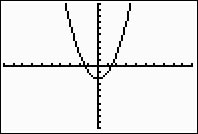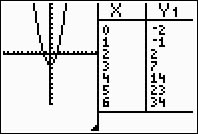SET CLOCK:   The clock allows you to set the time, date, and clock display.

 MathPrint mode adds a second MODE page:n/d  U n/d:   fractions displayed as an improper fraction OR as a mixed number.
 GO TO FORMAT GRAPH:   Selecting YES will automatically go to the FORMAT screen. The YES will not lock.FORMAT
 From STAT CALC: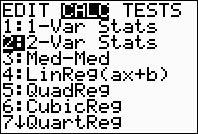you now see ...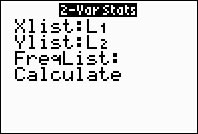Note: For most situations, leave FreqList blank. FreqList accepts list names only! When left blank, the frequency is assumed to be 1. After choosing Calculate (ENTER), you see ...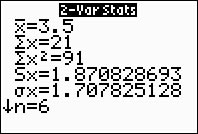This is a temporary results screen. Pressing CLEAR will return to a blank HOME screen. If you need to see the results again, arrow up on the HOME screen and press ENTER.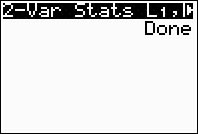Note that lines in MathPrint mode scroll left and right. Lines in Classic mode wrap on the screen. From STAT CALC: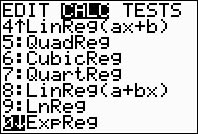you now see ...Note: For most situations, leave FreqList blank. FreqList accepts list names only! When left blank, the frequency is assumed to be 1. Include Y1 (or other Y location) as the Store RegEQ. Shortcut for Y1 is ALPHA, F4, ENTER.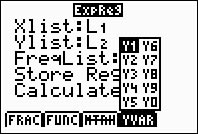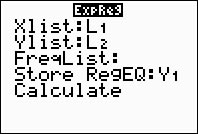After choosing Calculate (ENTER), you see ...This is a temporary results screen. Press GRAPH to see the plot and the regression equation.